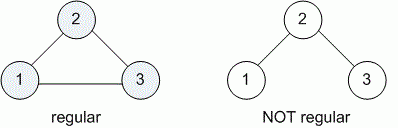Problems

# Regular graph

Time limit 1 second
Memory limit 128 MiB

Undirected graph is called regular, if all its vertices have the same degree.

Graph is given by list of edges. Check, is it regular.

## Input data

First line contains number n (1n100) of vertices and number m (mn (n - 1) / 2) of edges in a graph. Then given m pairs of numbers - the edges of graph.

## Output data

Print YES if graph is regular and NO otherwise.## Examples

Input example #1
3 3
1 2
1 3
2 3

Output example #1
YES

Input example #2
3 2
1 2
2 3

Output example #2
NO